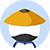The numbers D, N, and P are positive integers, such that D < N, and N is not a power of any other positive integer. Is D a prime number?

Statement #1: N has exactly four factors, and D is a factor of N

Statement #2: $D = (3^P) + 2$
• AStatement 1 ALONE is sufficient to answer the question, but statement 2 alone is NOT sufficient.
• BStatement 2 ALONE is sufficient to answer the question, but statement 1 alone is NOT sufficient.
• CBOTH statements 1 and 2 TOGETHER are sufficient to answer the question, but NEITHER statement ALONE is sufficient.
• DEach statement ALONE is sufficient to answer the question.
• EStatement 1 and 2 TOGETHER are NOT sufficient to answer the question. Previous

• 网友解析

### 题目讨论

• 优质讨论
• 最新讨论• 所属科目:数学DS
• 题目来源1:Magoosh-229
• 正确率: 27%
• 难度: